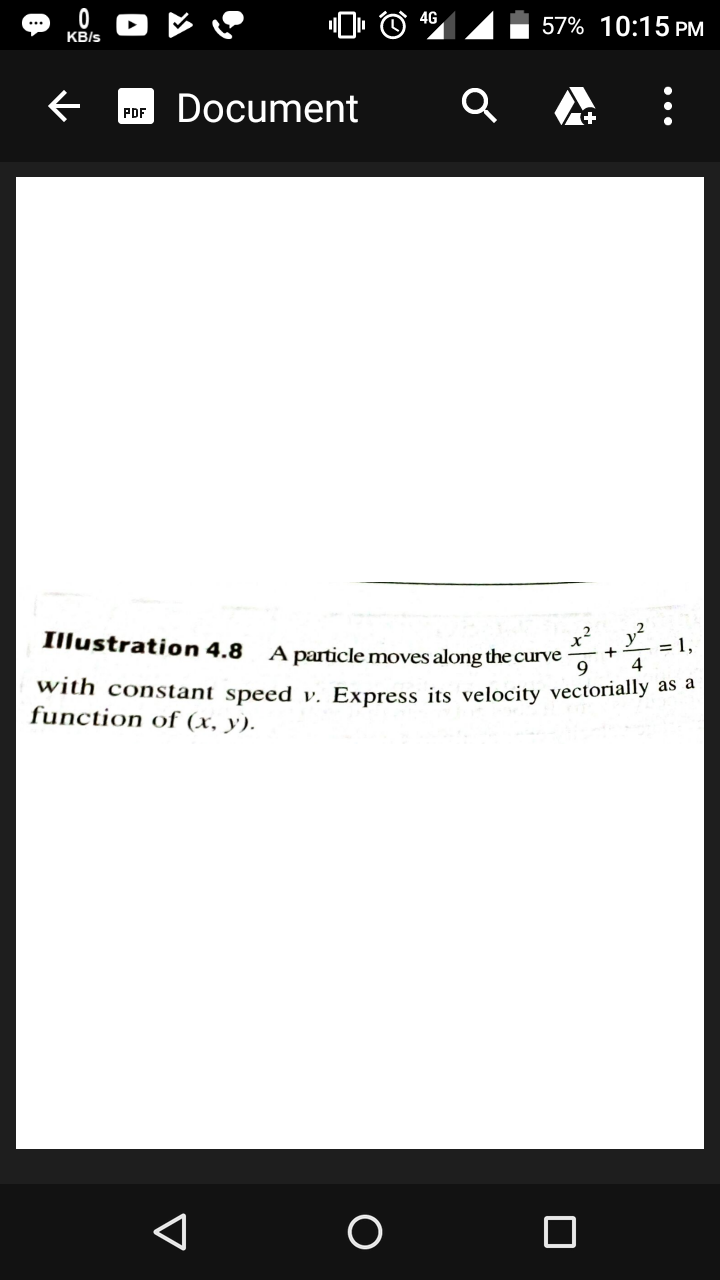#### Thank you for registering.

One of our academic counsellors will contact you within 1 working day.

Please check your email for login details.

Click to Chat

1800-1023-196

+91 7353221155

CART 0

• 0
MY CART (5)

Use Coupon: CART20 and get 20% off on all online Study Material

ITEM
DETAILS
MRP
DISCOUNT
FINAL PRICE
Total Price: Rs.

There are no items in this cart.
Continue Shopping

# A particle moves along the curve x^2/9+x^2/4=1 with constant speed v.express its velocity vectorially as a function of(x,y)## 1 Answers

2 years ago

So, effectively, the displacement of the particle with respect to the reference co-ordinate axes is:-

s= (x^2)/9 + (y^2)/4 =1.

Now, velocity is ds/dt.

So, we partially differentiate the equation, first with respect to X, treating you as a constant. This gives the velocity of the particle. (Vx) in X direction.

Vx= (2/9)x (i)^

Similarly, to get Vy, partially differentiate with respect to y.

Vy= y/2 (j)^

So, the velocity vector is

V= (2/9)X (i)^ + y/2 (j)^.

## ASK QUESTION

Get your questions answered by the expert for free